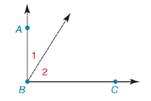Chapter 1.5, Problem 31EElementary Geometry For College St...

7th Edition
Alexander + 2 others
ISBN: 9781337614085

Solutions

Chapter
SectionElementary Geometry For College St...

7th Edition
Alexander + 2 others
ISBN: 9781337614085
Textbook Problem

In Exercises 27 to 35, complete the formal proof of each theorem.If the exterior sides of two adjacent acute angles form perpendicular rays, then these angles are complementary. Given: B A → ⊥ B C → Prove: ∠ 1 is complementary to ∠ 2PROOF Statements Reasons 1. B A → ⊥ B C → 1. ? 2. ? 2. If two rays are ⊥ , then they meet to form a right ∠ 3. m ∠ A B C = 90 ° 3. ? 4. m ∠ A B C = m ∠ 1 + m ∠ 2 4. ? 5. m ∠ 1 + m ∠ 2 = 90 ° 5. Substitution 6. ? 6. If the sum of the measures of two angles is 90 ° , then the angles are complementary

To determine

To find:

The complete formal proof of the given theorem.

Explanation

Given:

The statement of given theorem is,

If the exterior sides of two adjacent acute angles form perpendicular rays, then these angles are complementary.

Given: BABC

Prove: 1 is complementary to 2

The given figure is,

Definition:

If the sum of two angles is 90° then the angles are known as complementary angles.

Approach:

The formal proof of given theorem is explained in table below.

 Statements Reasons 1. BA→⊥BC→ 1. Given 2. ∠ABC is a right angle 2. If two rays are ⊥, then they meet to form a right ∠ 3. m∠ABC=90° 3. The measure of a right angle is 90°. 4. m∠ABC=m∠1+m∠2 4. Angle-addition postulate 5. m∠1+m∠2=90° 5

Still sussing out bartleby?

Check out a sample textbook solution.

See a sample solution

The Solution to Your Study Problems

Bartleby provides explanations to thousands of textbook problems written by our experts, many with advanced degrees!

Get Started

In Exercises 1124, find the indicated limits, if they exist. 23. limx3x2+2x+42x23x+1

Applied Calculus for the Managerial, Life, and Social Sciences: A Brief Approach

If P=2a+b,a=36,andb=15,findP.

Elementary Technical Mathematics

In Exercises 9-16, find the steady state vector for the transition matrix. 

Finite Mathematics for the Managerial, Life, and Social Sciences

The graph at the right is the direction field for: a) y = x y b) y = xy c) y = x + y d) y = xy

Study Guide for Stewart's Single Variable Calculus: Early Transcendentals, 8th